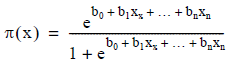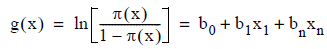# 5.4.5 - Logit Model - Teradata Warehouse Miner

## Teradata Warehouse Miner User Guide - Volume 3Analytic Functions

Product
Release Number
5.4.5
Published
February 2018
Language
English (United States)
Last Update
2018-05-04
dita:mapPath
yuy1504291362546.ditamap
dita:ditavalPath
ft:empty
The logit transformation function is chosen because of its mathematical power and simplicity, and because it lends an intuitive understanding to the coefficients eventually created in the model. The following equations describe the logistic regression model, with π(x) being the probability that the dependent variable is 1, and g(x) being the logit transformation:Notice that the logit transformation g(x) has linear parameters (b- values) and may be continuous with unrestricted range. Using these functions, a binomial error distribution is found with y = π(x) + Ɛ. The solution to a logistic regression model is to find the b- values that “best” predict the dichotomous y variable based on the values of the numeric x variables.